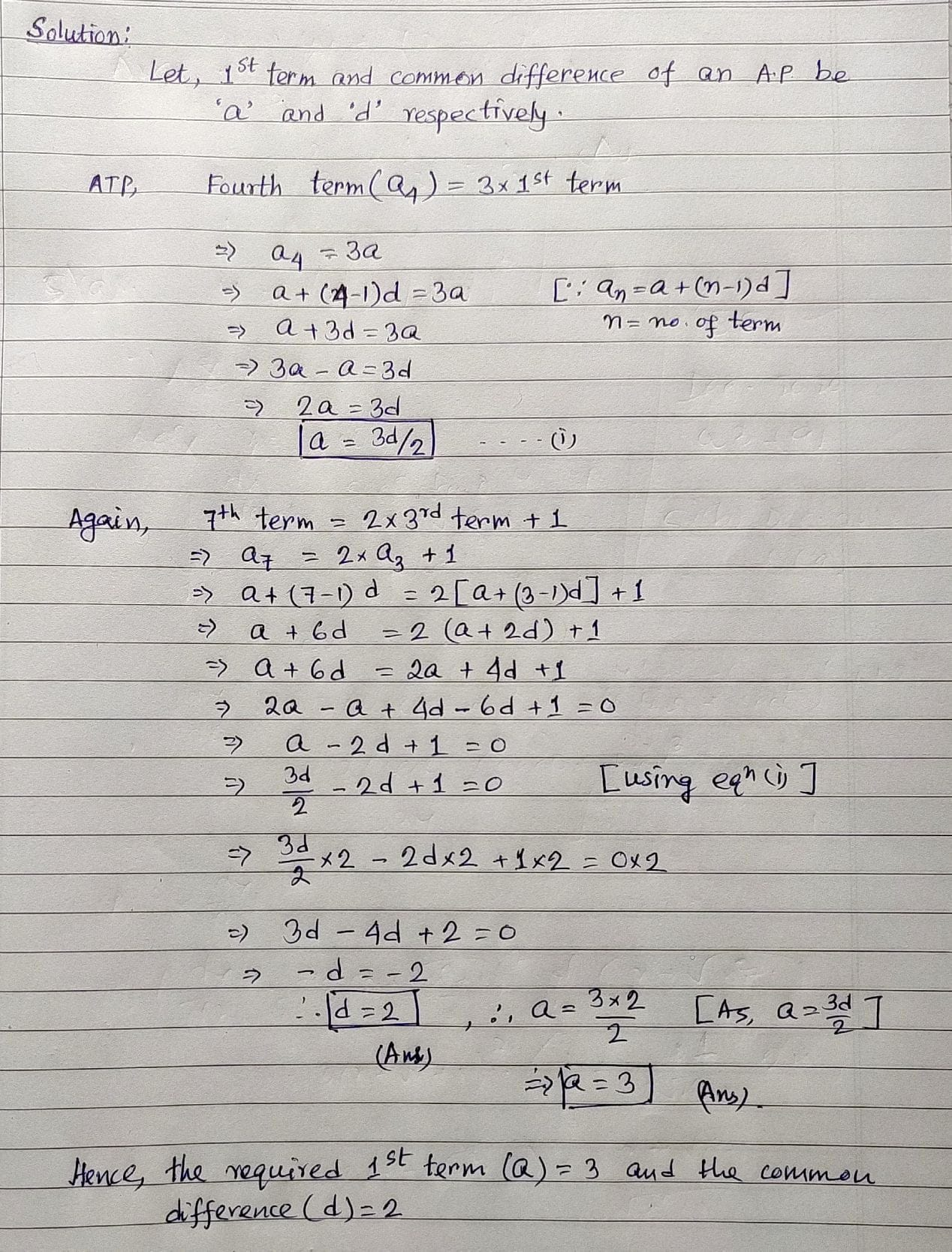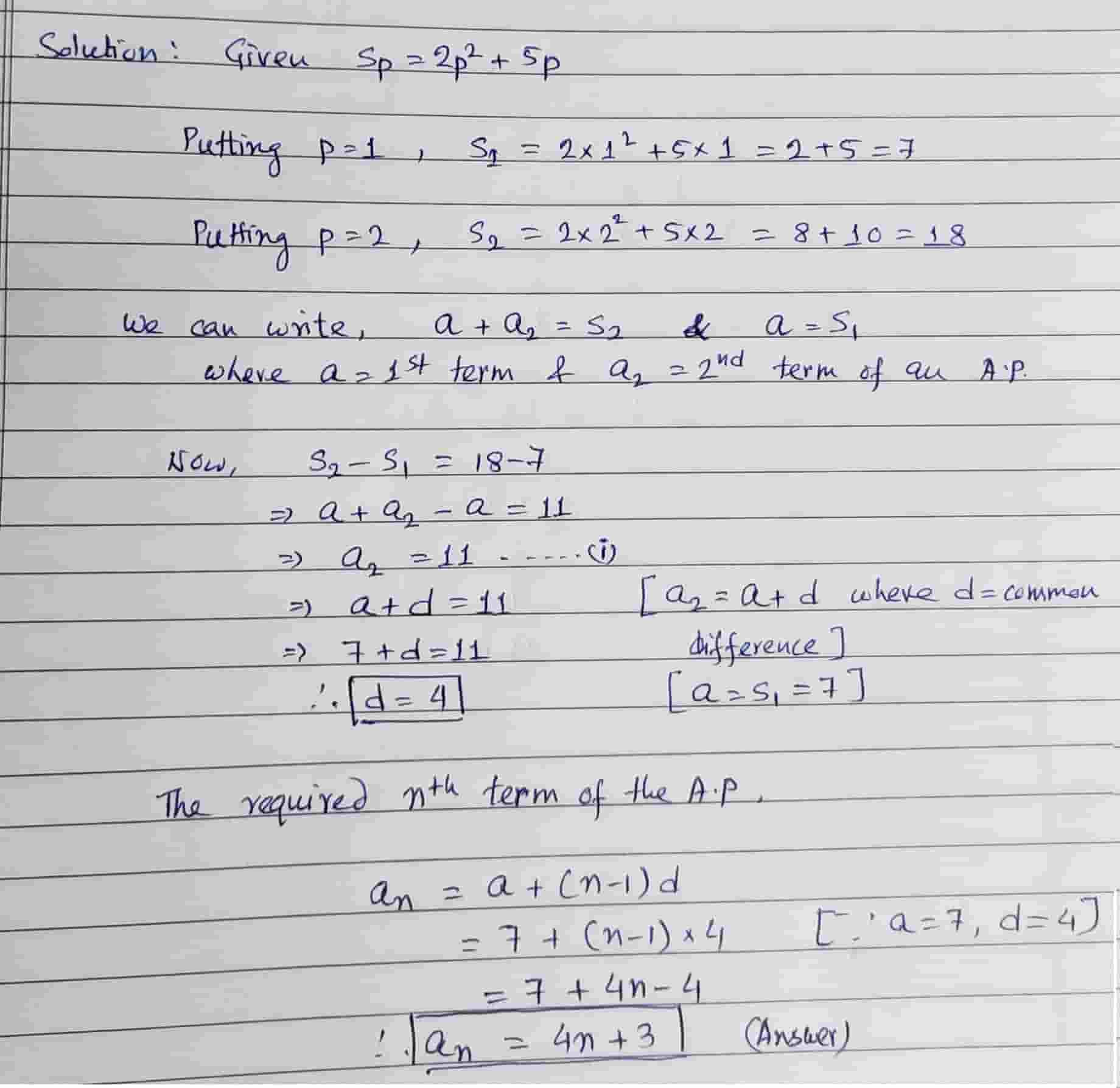Tue. Sep 26th, 2023

Aug 20, 2022

# BCS-012 Guess Paper for TEE June 2022

## BCS-012 Guess Paper for TEE June 2022

### Block 1: Algebra – I

#### Unit 1: Determinants

Q1. Show that  .

Q2. Show that

Q3. Show that

Q4. Solve the following system of linear equations using Cramer’s rule.

x + 2yz = 1

3x + 8y + 2z = 28

4x + 9y + z = 14

Q5. Find the area of the triangle with vertices at (−k + 1, 2k), (k, 2 − 2k) and (−4 − k, 6 − 2k). For what values of  k  these points are collinear ?

#### Unit 2: Matrices – I

Q1. Construct a  2×2  matrix  A = [aij] 2×2 where elements are given by  aij = (i + 2j)2.

Q2. If    and  f(x) = x2 –4x + 7,  then show that  f(A) = O2×2. Use this result to find  A5.

Q3. If  , then prove that  A2– 4A – 5I3 = O. Hence, obtain  A–1.

Q4. If    and   are two square matrices, verify that  AB = BA = 6I3. Hence, solve the system of linear equations :

xy = 3

2x + 3y + 4z = 17

y + 2z = 7.

#### Unit 3: Matrices – II

Q1. Reduce the matrix    to its normal form and hence determine its rank.

Q2. Reduce the matrix     to normal form by elementary operations.

Q3. Using elementary row operations find the inverse of the matrix .

#### Unit 4: Mathematical Induction

Q1. Use the principle of mathematical induction to prove that    for every natural number  n.

Q2. Show that  11  divides  102n–1 + 1  for every natural number  n.

### Block 2: Algebra – II

#### Unit 1: Sequence and Series

Q1. The fourth term of an arithmetic progression is equal to 3 times the first term and the seventh term exceeds twice the third term by 1. Find its first term and the common difference.Q2. If the sum of the first  p  terms of an A.P. is given by  Sp = 2p2 + 5p, Find the  nth  term of the A.P.Q3. If in an A.P.  a = 2 and the sum of first five terms is one-fourth of the sum of the next five terms, show that a20 = – 112.

Q4. The common ratio of a GP is  –4/5  and the sum to infinity is  80/9. Find the first term of the GP.

Q5. Find the sum to  n  terms of the series
0.6 + 0.66 + 0.666 + …………………..

#### Unit 2: Complex Number

Q1. If  ,  then show that    .

Q2. Use De Moivre’s theorem to find  (√3 + 1)3.

Q3. If  x = a + b, y = aω + 2  and  z = aω2 +  ,  show that  xyz = a3 + b3

#### Unit 3: Equations

Q1. If one root of the quadratic equation  ax2 + bx + c = 0 is square of the other. Prove that  b3 + a2c + ac2 = 3abc.

Q2. If  α, β  are the roots of the equation  2x2– 3x + 1 = 0  form an equation whose roots are  α/β  and  β/α.

Q3. Solve the equation  x4 − 2x3 + 4x2 + 6x − 21 = 0  being given that one root exceeds the other by 2.

Q4. Solve the equation  x4 + 2x3 − 21x2 − 22x + 40 = 0  given that sum of two of the roots being equal to the sum of the other two.

#### Unit 4: Inequalities

Q1. Solve the inequality    and graph its solution.

Q2. Solve the inequality    and graph its solution.

### Block 3: Calculus

#### Unit 1: Differential Calculus

Q1. Find     if  .

Q2. Find     if   .

Q3. If  y = aemx + be−mx , show that

#### Unit 2: Simple Application of Differential Calculus

Q1. A young child is flying kite which is at a height of 50 m. The wind is carrying the kite horizontally away from the child at a speed of 6.5 m/s. How fast must the kite string be let out when the string is 130 m ?

Q2. A man 180 cm tall walks at a rate of 2 m/s away from a source of light that is 9 m above the ground. How fast is the length of his shadow increasing when he is 3 m away from the base of light ?

Q3. Find the points of local maxima and minima, if any, of the following function

xR.

Find also the local maximum values and local minimum values.

Q4. Show that    has a local maximum at  .

Q1. Evaluate

Q2. Evaluate  .

Q3. Evaluate

#### Unit 4: Applications of Integral Calculus

Q1. Find the area bounded by the curves  y = xand  y = x.

Q2. Find the area bounded by the  x-axis,  y = 2 + 3x  and the ordinates  x = 0  and  x = 3.

Q3. Find the length of the curve    from (0, 3) to (2, 4).

### Block 4: Vectors and Three-Dimentional (3-D) Geometry

#### Unit 1: Vectors – 1

Q1. If the mid-points of the consecutive sides of a quadrilateral are joined, then show by using vectors that they form a parallelogram.

Q2. Show that the three points : A(6, –7, –1), B(2, –3, 1) and C(4, –5, 0) are collinear.

#### Unit 2: Vectors – 2

Q1. Find the area of ABC with vertices A (1, 3, 2), B (2, –1, 1)  and  C(–1, 2, 3).

Q2. Find a unit vector perpendicular to each of the vector   and  , where    and

Q3. Find the value of  λ  for which the vectors   and   are coplanar.

#### Unit 3: Three-Dimentional Geometry – 1

Q1. Show that the lines     and     intersect.

Q2. Find the shortest distance between the lines   and  .

#### Unit 4: Linear Programming

Q1. Find the maximum value of  5x + 2y  subject to the constraints

– 2x – 3y ≤ –6
x – 2y ≤ 2
6x + 4y ≤ 24
– 3x +2y ≤ 3
x ≥ 0, y ≥ 0

Q2. If a young man rides his motor cycle at 25 km per hour, he has to spend Rs. 2 per km on petrol; if he rides it at a faster speed of 40 km per hour, the petrol cost increases to Rs. 5 per km. He wishes to spend at most Rs. 100 on petrol and wishes to find what is maximum distance he can travel within one hour. Express this as a linear programming problem and then solve it.

Q3. A manufacturer wishes to produce two types of steel trunks. He has two machines A and B. For completing, the first type of trunk, he requires 3 hrs on machine A and 2 hrs on machine B whereas the second type of trunk requires 3 hrs on machine A and 3 hrs on machine B. Machines A and B can work at the most for 18 hrs and 14 hrs per day respectively. He earns a profit of Rs. 30 and Rs. 40 per trunk of first type and second type respectively. How many trunks of each type must he make each day to make maximum profit? What is his maximum profit?

#### Navigating JENPAUH 2021: Your Gateway to a Bright Medical Career

error: Content is protected !!